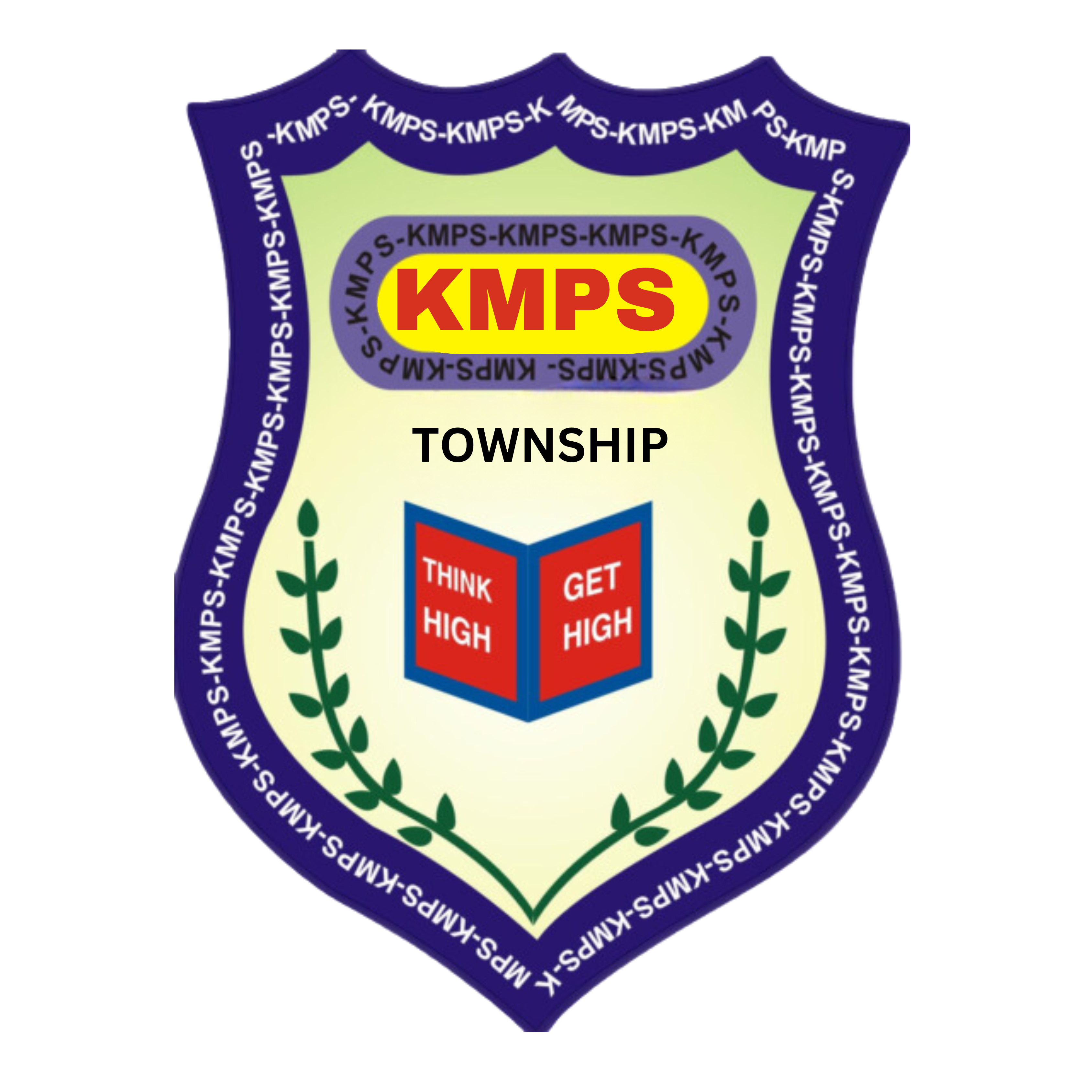Kanha Makhan Public School

Infront of Radha Town,NH-2, Refinery, Township, Mathura

MATHS LABMath needs practice, interest and brainpower which are fostered by the Math lab. The KMPS Math Lab makes Math simple and fun for students. The lab is well equipped to demonstrate any topic. It also enables the teachers to reinforce Mathematical concepts. Hence, it helps in mental enhancement of students in any area of Math like Algebra, Arithmetic, Calculus, Geometry, Statistics, and Trigonometry etc.